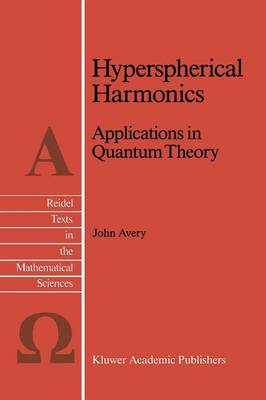Find your perfect holiday reading•# Hyperspherical Harmonics: Applications in Quantum Theory - Reidel Texts in the Mathematical Sciences 5 (Paperback)

(author)
£139.99
Paperback 256 Pages / Published: 21/01/2012
• We can order this

Usually dispatched within 3 weeks

• This item has been added to your basket
where d 3 3)2 ( L x - -- i3x j3x j i i>j Thus the Gegenbauer polynomials play a role in the theory of hyper spherical harmonics which is analogous to the role played by Legendre polynomials in the familiar theory of 3-dimensional spherical harmonics; and when d = 3, the Gegenbauer polynomials reduce to Legendre polynomials. The familiar sum rule, in 'lrlhich a sum of spherical harmonics is expressed as a Legendre polynomial, also has a d-dimensional generalization, in which a sum of hyper spherical harmonics is expressed as a Gegenbauer polynomial (equation (3-27": The hyper spherical harmonics which appear in this sum rule are eigenfunctions of the generalized angular monentum 2 operator A , chosen in such a way as to fulfil the orthonormality relation: VIe are all familiar with the fact that a plane wave can be expanded in terms of spherical Bessel functions and either Legendre polynomials or spherical harmonics in a 3-dimensional space. Similarly, one finds that a d-dimensional plane wave can be expanded in terms of HYPERSPHERICAL HARMONICS xii "hyperspherical Bessel functions" and either Gegenbauer polynomials or else hyperspherical harmonics (equations ( 4 - 27) and ( 4 - 30) ) : 00 ik*x e = (d-4)!!A~oiA(d+2A-2)j~(kr)C~(~k'~) 00 (d-2)!!I(0) 2: iAj~(kr) 2:Y~ (["2k)Y (["2) A A=O ). l). l)J where I(O) is the total solid angle. This expansion of a d-dimensional plane wave is useful when we wish to calculate Fourier transforms in a d-dimensional space.

Publisher: Springer
ISBN: 9789401075442
Number of pages: 256
Weight: 427 g
Dimensions: 235 x 155 x 14 mm
Edition: Softcover reprint of the original 1st ed. 198

MEDIA REVIEWS
`Since the book is clearly written, it will be useful to students and research workers in mathematics, nuclear physics, atomic physics and quantum chemistry.'
G. van den Berghe in Mathematical Reviews

## Reviews

Sign In To Write A Review

Please sign in to write a review

Your review has been submitted successfully.